# PSAT Math : How to simplify an expression

## Example Questions

### Example Question #44 : Expressions

Increase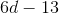by 70%. Which of the following will this be equal to?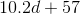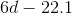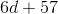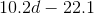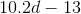Explanation:

A number increased by 70% is equivalent to 100% of the number plus 70% of the number. This is taking 170% of the number, or, equivalently, multiplying it by 1.7.

Therefore,increased by 70% is 1.7 times this, or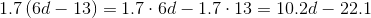### Example Question #45 : Expressions

Which of the following phrases can be written as the algebraic expression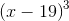?

Three times the difference of a number and nineteen

The cube of the difference of nineteen and a number

Nineteen less than the cube of a number

Three times the difference of nineteen and a number

The cube of the difference of a number and nineteen

The cube of the difference of a number and nineteen

Explanation:is the cube of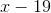, which is the difference of a number and nineteen; therefore,is "the cube of the difference of a number and nineteen."

### Example Question #46 : Expressions

Which of the following phrases can be written as the algebraic expression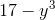?

The cube of the difference of a number and seventeen

Seventeen decreased by the cube of a number

Three times the difference of seventeen and a number

Three times the difference of a number and seventeen

The cube of the difference of seventeen and a number

Seventeen decreased by the cube of a number

Explanation:is seventeen decreased by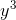, which is the cube of a number; therefore,is "seventeen decreased by the cube of a number."

### Example Question #47 : Expressions

Which of the following operations could represent the expression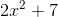?

7 less than the square of 2x

7 more than 2 times the square of x

2 times 7 more than the square of x

2 times 7 less than the square of x

7 more than the square of 2x

7 more than 2 times the square of x

Explanation:

Begin by putting the equation given into your own words. It might sound something similar to:

2 times x squared plus 7

Now, go through each answer choice and see if any of them are similar to this. We immediately see that the answer "7 more than 2 times the square of x" is similar to what we came up with. Let's do a quick run through of the other choices to be sure of our choice:

"2 times 7 more than the square of x" is equal to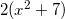, which is equivalent to"2 times 7 less than the square of x" is equal to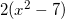, which is equivalent to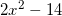.

"7 more than the square of 2x" is equal to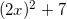, which is equivalent to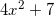.

"7 less than the square of 2x" is equal to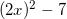, which is equivalent to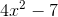.

The only answer that works is "7 more than 2 times the square of x". This is the correct answer.

### Example Question #48 : Expressions

What does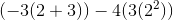equal?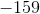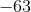Explanation:

When solving a complex expression, remember the acronym PEMDAS (Parentheses, Exponents, Multiplication, Division, Addition, Subtraction). Reading left to right, begin by doing all operations within the innermost Parentheses first: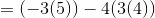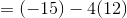Continue simplifying using the acronym PEMDAS: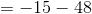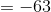The expression is equal to -63.